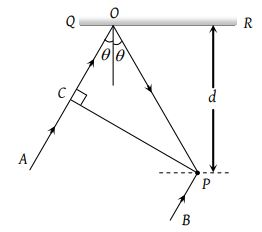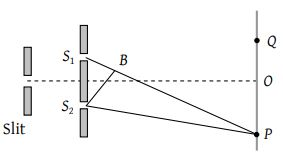In the visible region of the spectrum the rotation of the place of polarization is given by $\theta =a+\frac{b}{{\lambda }^{2}}$. The optical rotation produced by a particular material is found to be 30° per mm at $\lambda =5000$Å and 50° per mm at $\lambda =4000\text{Å}$. The value of constant a will be

(1) $+\frac{50°}{9}$per mm

(2) $-\frac{50°}{9}$per mm

(3) $+\frac{9°}{50}$per mm

(4) $-\frac{9°}{50}$per mm

Concept Questions :-

Polarization of light
High Yielding Test Series + Question Bank - NEET 2020

Difficulty Level:

When an unpolarized light of intensity I0 is incident on a polarizing sheet, the intensity of the light which does not get transmitted is

(1) Zero

(2) I0

(3) $\frac{1}{2}{I}_{0}$

(3) $\frac{1}{4}{I}_{0}$

Concept Questions :-

Polarization of light
High Yielding Test Series + Question Bank - NEET 2020

Difficulty Level:

When the angle of incidence on a material is 60°, the reflected light is completely polarized. The velocity of the refracted ray inside the material is (in ms–1)

(1) 3 × 108

(2) $\left(\frac{3}{\sqrt{2}}\right)×{10}^{8}$

(3) $\sqrt{3}×{10}^{8}$

(4) 0.5 × 108

Concept Questions :-

Polarization of light
High Yielding Test Series + Question Bank - NEET 2020

Difficulty Level:

Two polaroids are placed in the path of unpolarized beam of intensity I0 such that no light is emitted from the second polaroid. If a third polaroid whose polarization axis makes an angle θ with the polarization axis of first polaroid, is placed between these polaroids then the intensity of light emerging from the last polaroid will be

(1) $\left(\frac{{I}_{0}}{8}\right){\mathrm{sin}}^{2}2\theta$

(2) $\left(\frac{{I}_{0}}{4}\right){\mathrm{sin}}^{2}2\theta$

(3) $\left(\frac{{I}_{0}}{2}\right){\mathrm{cos}}^{4}\theta$

(4) ${I}_{0}{\mathrm{cos}}^{4}\theta$

Concept Questions :-

Polarization of light
High Yielding Test Series + Question Bank - NEET 2020

Difficulty Level:

In the adjacent diagram, CP represents a wavefront and AO & BP, the corresponding two rays. Find the condition on θ for constructive interference at P between the ray BP and reflected ray OP(1) cosθ = 3λ/2d

(2) cosθ = λ/4d

(3) secθ – cosθ = λ/d

(4) secθ – cosθ = 4λ/d

Concept Questions :-

Interference vs Diffraction
High Yielding Test Series + Question Bank - NEET 2020

Difficulty Level:

In the Young's double slit experiment, if the phase difference between the two waves interfering at a point is ϕ, the intensity at that point can be expressed by the expression-

(where A and B depend upon the amplitudes of the two waves)

(1) $I=\sqrt{{A}^{2}+{B}^{2}{\mathrm{cos}}^{2}\varphi }$

(2) $I=\frac{A}{B}\mathrm{cos}\varphi$

(3) $I=A+B\mathrm{cos}\frac{\varphi }{2}$

(4) $I=A+B\mathrm{cos}\varphi$

Concept Questions :-

Young Double slit experiment
High Yielding Test Series + Question Bank - NEET 2020

Difficulty Level:

When one of the slits of Young’s experiment is covered with a transparent sheet of thickness 4.8 mm, the central fringe shifts to a position originally occupied by the 30th bright fringe. What should be the thickness of the sheet if the central fringe has to shift to the position occupied by 20th bright fringe

(1) 3.8 mm

(2) 1.6 mm

(3) 7.6 mm

(4) 3.2 mm

High Yielding Test Series + Question Bank - NEET 2020

Difficulty Level:

In the ideal double-slit experiment, when a glass-plate (refractive index 1.5) of thickness t is introduced in the path of one of the interfering beams (wavelength λ), the intensity at the position where the central maximum occurred previously remains unchanged. The minimum thickness of the glass-plate is

(1) 2λ

(2) $\frac{2\lambda }{3}$

(3) $\frac{\lambda }{3}$

(4) λ

High Yielding Test Series + Question Bank - NEET 2020

Difficulty Level:

In the figure is shown Young’s double slit experiment. Q is the position of the first bright fringe on the right side of O. P is the 11th fringe on the other side, as measured from Q. If the wavelength of the light used is 6000 × 10–10 m, then S1B will be equal to(1) 6 × 10–6 m

(2) 6. 6 × 10–6 m

(3) 3.138 × 10–7 m

(4) 3.144 × 10–7 m

Concept Questions :-

Young Double slit experiment
High Yielding Test Series + Question Bank - NEET 2020

Difficulty Level:

In Young’s double slit experiment, the two slits act as coherent sources of equal amplitude A and wavelength λ. In another experiment with the same set up the two slits are of equal amplitude A and wavelength λ but are incoherent. The ratio of the intensity of light at the mid-point of the screen in the first case to that in the second case is

(1) 1 : 2

(2) 2 : 1

(3) 4 : 1

(4) 1 : 1

Concept Questions :-

Young Double slit experiment